Disclaimer: This is an example of a student written essay.

Any opinions, findings, conclusions or recommendations expressed in this material are those of the authors and do not necessarily reflect the views of UKEssays.com.

# Visual Interpretation of Stock Prediction Using Sentiment Analysis

 ✅ Paper Type: Free Essay ✅ Subject: Computer Science ✅ Wordcount: 12050 words ✅ Published: 18th May 2020

VISUAL INTERPRETATION OF STOCK PREDICTION USING SENTIMENT ANALYSIS

Abstract

Introduction

Stock markets can play a pivotal role in stabilizing the economies on a global scale by avoiding the repercussions of a recession by evading the potential bankruptcy of companies and loss of billions for the investors and shareholders and thus avoiding the collapse of stock markets(“Importance of stock market”,n.d.). The stockholders and traders follow many approaches and strategies to make better trading decisions.

Trading plan involves the prerequisites that one needs to have before planning anything in general. One needs to know the terminology, the nitty-gritty of the trading market. One must also know the reasoning behind the past gains or losses. Planning needs analyzing the situation and then a plan to deal with it.

Capital investment and protection. To start a trade the first step is to introduce the capital. Accumulation of it must have involved a lot of savings and effort along with time. One needs to be very vigilant as the losses can be to the extent of getting bankrupted. (folger j,2019)

The stock traders must be focused on learning more each day. Focused observation, analysis of the stock market trends are prerequisites. The nature of stock markets is very dynamic meaning that it depends on several factors including world politics, events, economies, oil supply/demand, world leader ideologies and even the weather.

Risking wisely. Risk must only be taken if you can afford to lose. The assets used for trading must be expendable and not be allotted for any other purposes. Because losing money can be traumatic.

The trading decisions must be made based on facts. We can always research regarding, on internet. And the risks of this could be internet thefts and false sources. One must know the Genuity of information and its sources.

It is also advisable to always reevaluate your trading plan due to the sudden stock market fluctuations and changing trends. It is also very important to put trading in perspective. That is one must not totally indulge their emotions with trade wins/losses. This will facilitate for a healthy stock trading.

Utilization of technology. Technology utilization is having the know-hows of viewing and analyzing the markets through internet. Getting updates through smart phones allows us to monitor trade prices, equities, bonds etc. virtually from anywhere. As discussed earlier the economy of most of the countries is dependent on the stock market trading, it has the potential to uplift the economy and collapse. Many machine learning technologies are now being used to predict the stock prices. Out of the many factors responsible for the stock price changes social media is playing a significant role. The news relating to an organization or the opinions/discussions held on twitter are playing a role in forming a biased view towards an organization. This may have a fair amount of effect on an organizations stock price. For example, the news of Steve jobs’s death. Here the investor will be in the fear of investing in the apple stocks so for a few days stocks for Apple Inc may be decrease in value . Another one, the headlines can directly affect the stock price of next day like US banning Huawei phones completely. This will affect next day stock price of Huawei people will be willing to sell the stock.

In this project I have taken up the new headlines relating to apple company and tried to predict the organizations future stock price changes through sentiment analysis.

Modules

The headlines regarding a company are downloaded every day and stored in the database. the psycopg2 api is used to set up a connection to the dbms. These headlines are downloaded from the Finviz and Nasdaq news websites. The postgres database management systems is used to store the data. This project is developed using the headlines from April 1st to June 27th, 2019. By going through Nasdaq news website the data taken is changed into month-date-year format (from month-date-year).  The required data is crawled through web and is stored as a dataframe in the database. This dataframe has the following fields: date, name(organization), exchange(Nasdaq), source(news websites),  headlines. Here is the table that represents this dataframe: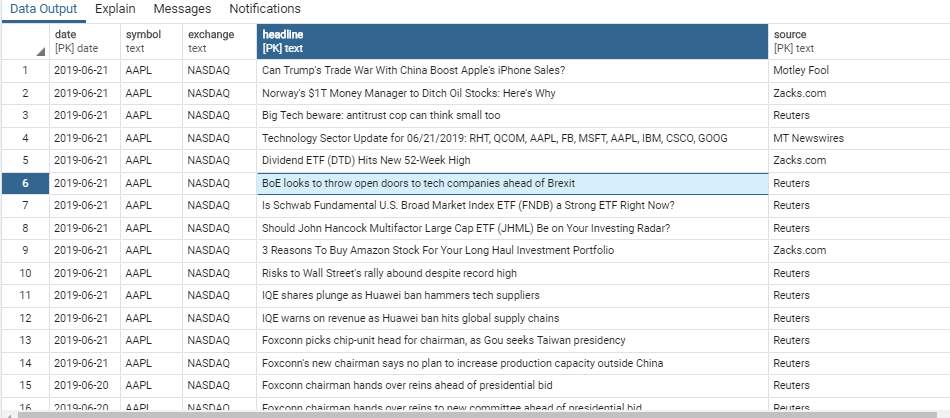Data Preprocessing

The headlines that are obtained from the news websites are preprocessed by removing special characters and numbers. It also contains a get_continuous_chunks(text) method which initially splits off the words in the sentence with its punctuation using word_tokenize() of nltk library. These words are then tagged with its parts of speech with the help of pos_tag(). The output of this is then passed onto the ne_chunk() which groups them into chunks. Tree functionality of nltk then represents them into a hierarchical grouping of leaves and sub trees. This method will return the entities that are present in each headline. this will facilitate for the user to easily search and identify for a headline by using the entities instead of skimming through the whole bunch of data. The dataframe representing the output of this function is stored in this way.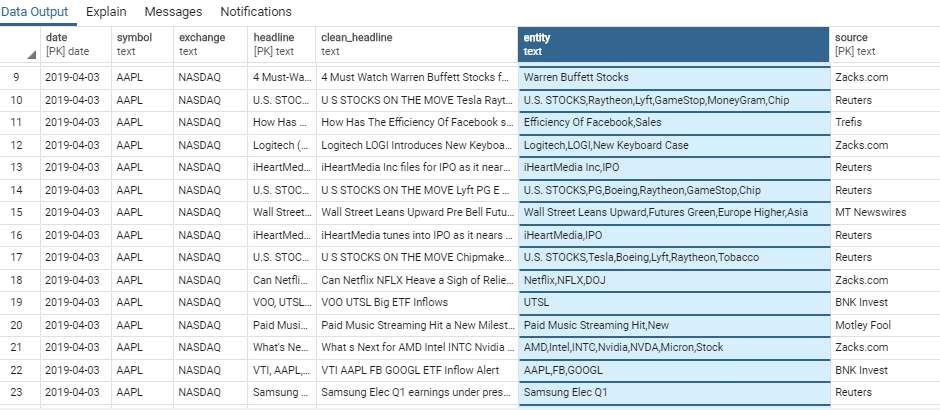Getting Polarity

So, polarity for each headline is generated using one of the three methods(simple polarity, textblob or Vader).

In simple polarity,  sentiment classification is done with Liu and Hu opinion lexicon. This function counts the number of positive, neutral and negative words in a sentence and classifies it on the basis of polarity of the headline. Words that are not in the lexicon are considered as neutral.(Pantane,n.d.)

def get_simple_polarity(sentence):

tokenizer = nltk.tokenize.treebank.TreebankWordTokenizer()

pos_words = 0

neg_words = 0

neutral = 0

tokenized_sent = [word.lower() for word in tokenizer.tokenize(sentence)]

if len(tokenized_sent) == 0:

return 0

opinion_lexicon = nltk.corpus.opinion_lexicon

for word in tokenized_sent:

if word in opinion_lexicon.positive():

pos_words += 1

elif word in opinion_lexicon.negative():

neg_words += 1

else:

neutral += 1

total_words = pos_words + neg_words + neutral

polarity = (pos_words – neg_words)/total_words

return polarity

####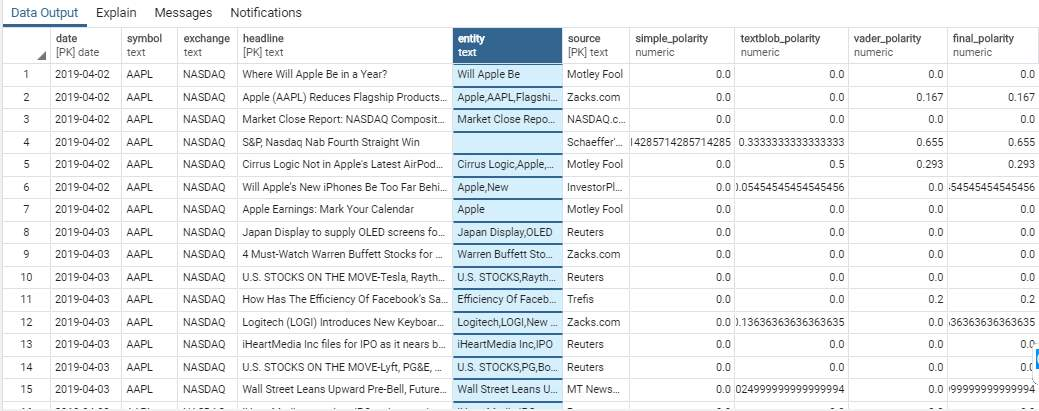Generating Sentiment Values

Basically, the function that calculates the sentiment for that day considers three parameters. One is the polarity of the present day then is the previous days sentiment value where day depends on which weekday it is, and the third parameter is the todays date. If it is not a Sunday, then its previous sentiment value will be the sentiment of the previous day. For example, if you want to calculate the sentiment of Monday then your previous sentiment will be of Sunday. If you want to calculate the sentiment of Sunday, then polarity of three days that is Friday Saturday and Sunday are considered. And the previous days sentiment will be the sentiment of Thursday. The reason for this is that the stock market is closed on weekends i.e. Saturday and Sunday. So, while predicting stock price changes for Monday, the headlines on Friday, Saturday and Sunday are considered.

If you need assistance with writing your essay, our professional essay writing service is here to help!

The recent polarity obtained is taken and the count of the positive, negative and neutral is stored in respective variables( pos_count, neg_count, neutral_count). The mean of these count variables is taken. For the neutral polarities to not normalize the mean they are not considered. If mean by any chance is not a number, then it is assigned a value of 0.

By using the EMA(Exponential Moving averages) method we calculate 10 sentiment values by giving varying weightages to previous sentiment and the mean we procured. the tenth value will be the mean calculated. The alpha value varies from 0 to 1 in the intervals of 0.1. The formula used is given below:

ema = (1-alpha) * prev_sentiment + alpha * todays mean_value

final_df[‘p_10’] = 0.9 * sentiment_df[‘p_10’] + 0.1 * mean_value

In the above statement the previous sentiment is given a higher weightage which depict the long-term effects of a news headline on present stock prices.

Similarly

final_df[‘p_90’] = 0.1 * sentiment_df[‘p_90’] + 0.9 * mean_value

gives more importance to present day news headlines than the previous news.

The average of all such values is taken and that is the sentiment for that day.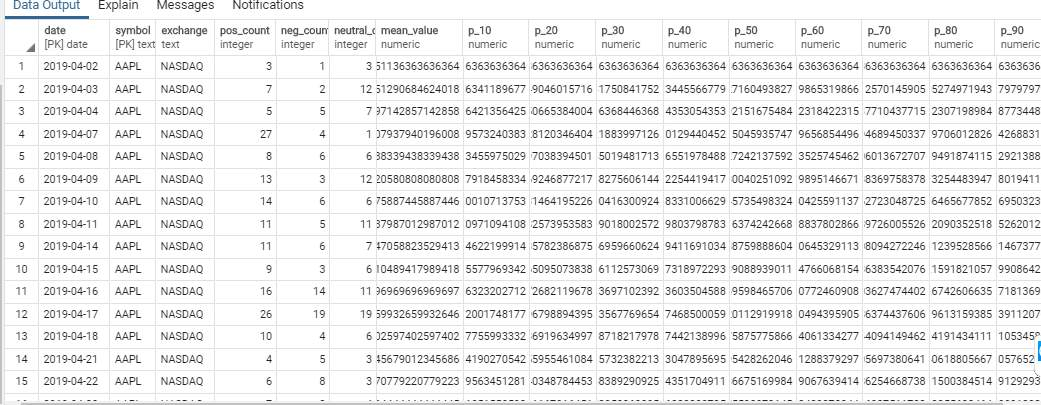Result Evaluation

This program recommends whether to buy or sell a stock by generating buy, sell, strong buy and  strong sell signals. A strong buy or strong sell signal is generated if the buy or sell percentages exceed or equals to 80%.the other cases are explained below with appropriate code. Even the intraday predictions are done. In intraday stock exchanges a stockholder wants to buy and sell the stock on almost the same day to incur small profits on the stock.

The recommendation value for a weekday is stored in its previous day’s recommendation value. For example, the recommendation for Wednesday is given by the recommendation value of Tuesday.

First, the stock prices of that day are stored into the database to get the close-open value which also helps determine the next day’s recommendation. The previous sentiment information for that day is extracted from the database. The tenth value is the mean value which considers only todays polarity not yesterday’s sentiment. the following conditions are considered to initially generate the buy/sell signals:

for i in range(6,16):

if rows[i] > 0 and change > 0 :

elif rows[i] > 0 and change < 0:

if rows[i] < 0 and change < 0 :

result.append(“sell”)

elif rows[i] < 0 and change > 0:

result.append(“sell”)

Here the rows[i] take the values from mean,p_10,p_20,p_30,…p_90. The above conditions are explained below:

• if sentiment value is positive and change in stock price is positive then buy signal is generated because the sentiment value favors the stocks and there already has been an increase in price the previous day.
• if sentiment value is positive and change in stock price is negative then buy signal is generated as the sentiment is in favor and stock price has decreased which further decreases the cost price.
• if sentiment value is negative and change in stock price is positive then sell signal is generated. As the stock you have may lose its price the next day and the change on that day positive indicating increase in stock that day.
• if sentiment value is negative and change in stock price is negative then sell signal is generated. The reason for this being that the stock has already decreased in value and on further delay would decrease it value furthermore.

A percentage of these buy/sell signals is calculated.

sell_percent = (int(result_dict[‘sell’])/10) * 100

      Then the following conditions are given to generate the final strong buy/sell, buy, sell signals for the next day.

      As the buy percentage is greater than or equal to 80% a strong buy signal is generated.

      A buy signal is generated as the buy percentage being less than 80 is still greater than the sell percentage for that day.

elif sell_percent >= 80.0:

final_prediction = ‘Strong Sell’

      As the sell percentage is greater or equals 80%.

elif sell_percent < 80.0 and sell_percent > buy_percent:

final_prediction = ‘Sell’

      a sell signal is generated as the sell percentage being less than 80 is still greater than the buy percentage for that day.

final_prediction = “Hold”

      hold signal also exists in cases where the buy and sell percentages are equal.

A dataframe with the values date, symbol,  exchange, open, high, low, close, change, mean, positive_count, negative_count, neutral_count, buy percentage, sell percentage and final prediction is stored  into the database.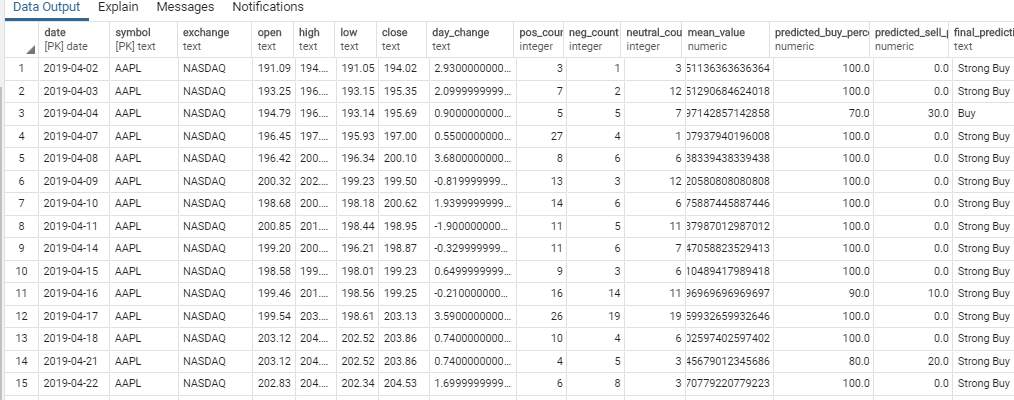generate_result_summary(date, stock_df, recommendation_df)

This method determines whether the recommendation for that day was accurate or not even for intraday stock trading. For this it needs the actual stock prices of that day.

For interday stock prediction accuracy it compares final prediction and (close-open) values. If the final prediction is buy or strong buy and the difference(close-open) is positive than our prediction is correct else wrong.

if close_open_change > 0:

result_interday = ‘Correct’

else:

result_interday = ‘Wrong’

And

if close_open_change > 0:

result_interday = ‘Correct’

else:

result_interday = ‘Wrong’

If the final prediction is sell or strong sell and the difference(close-open) is negative than our prediction is correct else wrong.

elif final_prediction == ‘Sell’ :

if close_open_change < 0:

result_interday = ‘Correct’

else:

result_interday = ‘Wrong’

elif final_prediction == ‘Strong Sell’ :

if close_open_change < 0:

result_interday = ‘Correct’

else:

result_interday = ‘Wrong’

The final prediction is compared with difference between high/low and open prices.

The final dataframe with all the new values is stored into the database as below:

This program checks for the accuracy of the prediction made by considering the actual  stock prices that are released when the stock market is closed for the day.

The counts of rightly and wrongly predicted values is taken, and percentage is taken.

correct_interday = len(df.loc[df[‘result_interday’] == ‘Correct’])

wrong_interday = len(df.loc[df[‘result_interday’] == ‘Wrong’])

total_interday = correct_interday + wrong_interday

accuracy_interday = (correct_interday/total_interday) * 100

The accuracy thus obtained for this project for interday prediction is  50% and intraday is 54.545454%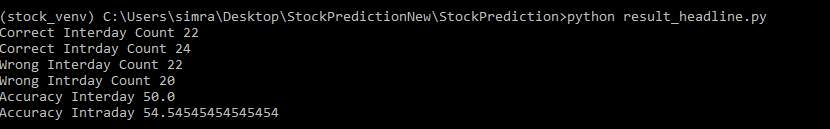Dashboard.py

I have programmed a dashboard using dash components in python to represent the results of the project. It contains tabs with livestock prices, pie chart, donut chart, bar graph and tables containing various findings.

The live prediction tab has the livestock prices that are represented with a line graph which gets updated after every five minutes. This tab also has a donut chart representing the buy and sell percentages for that day. Alongside this chart is the pie chart which represents the total number of positive, negative and neutral headlines percentage. The table at last represents the headlines with the polarity generated for them.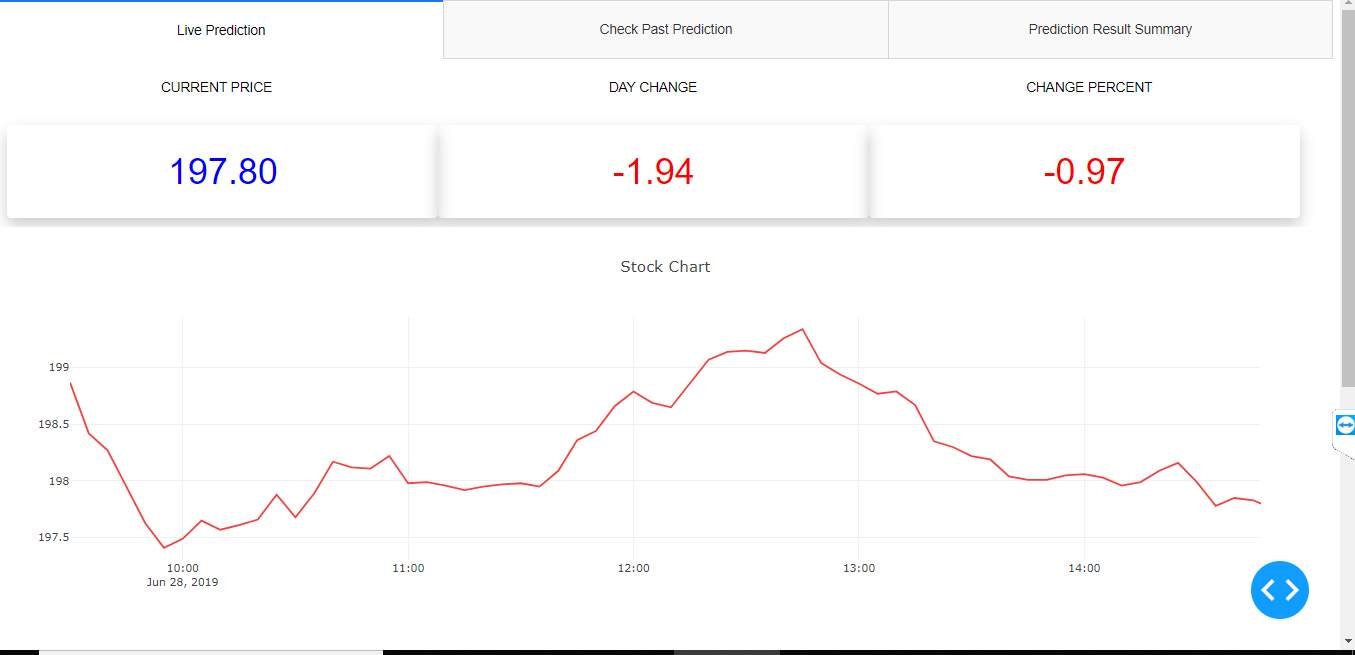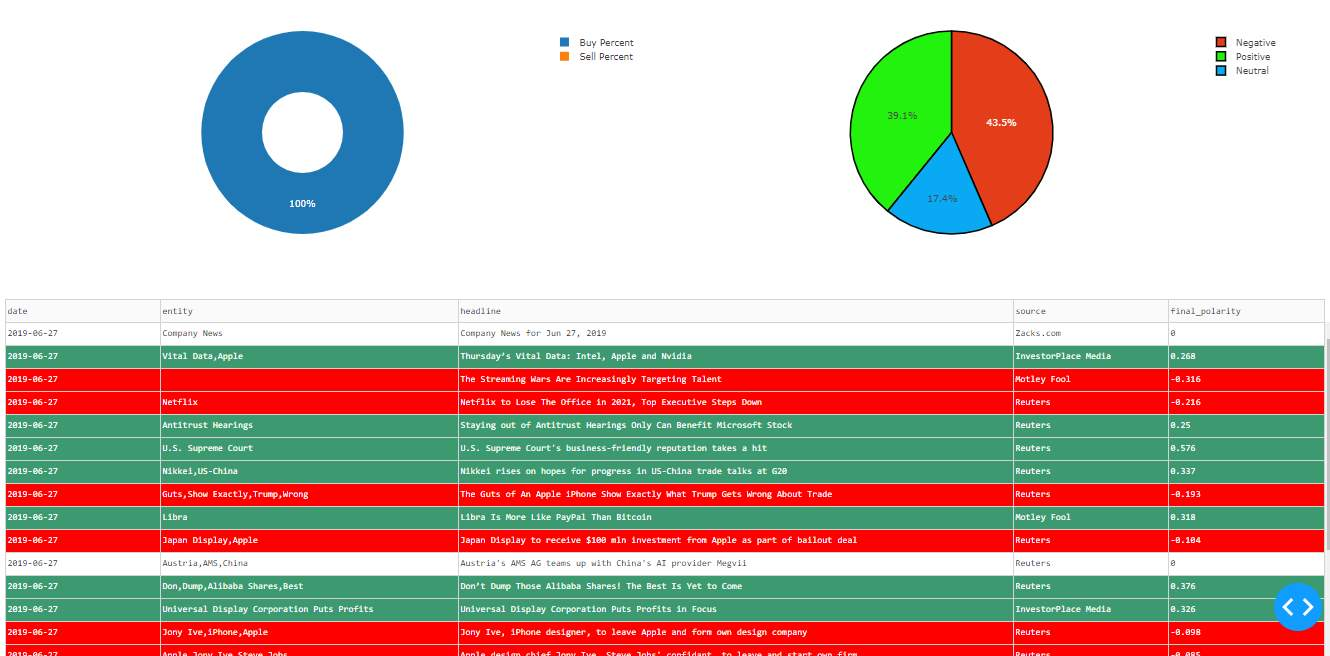The next tab represents the past day prediction details with the stock prices for time intervals of that day as a line graph. The donut and the pie charts represent the values of the past day.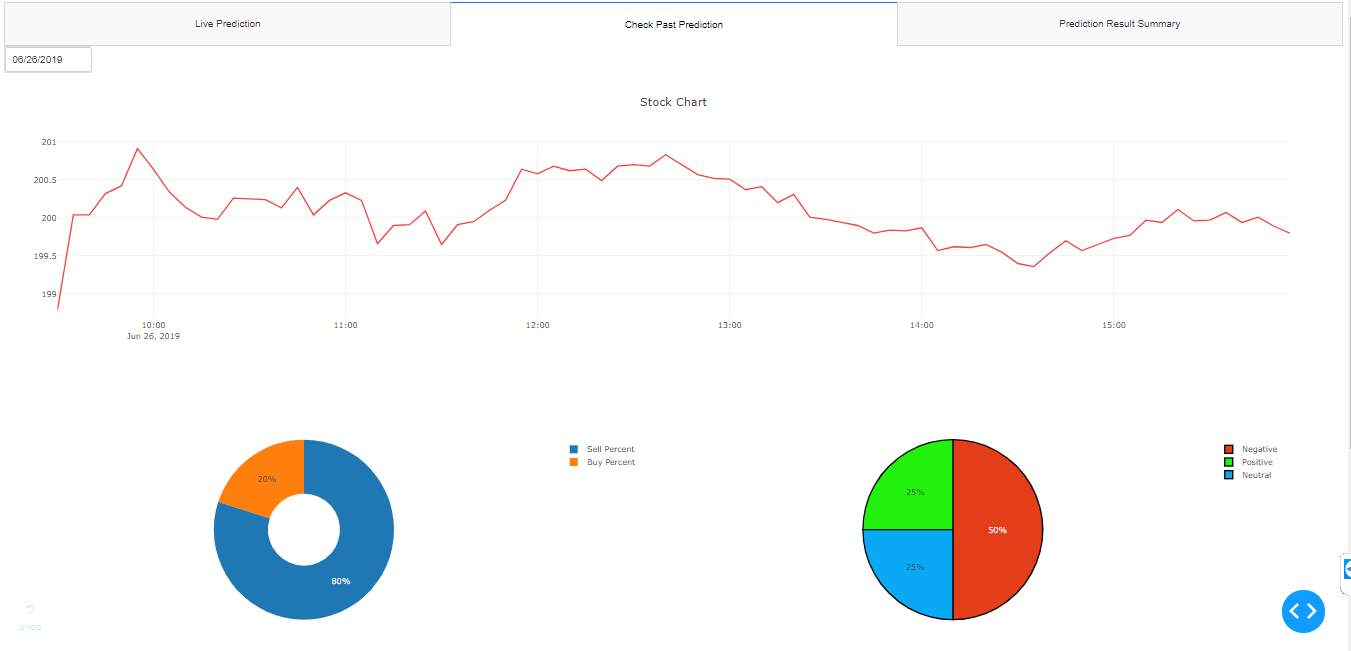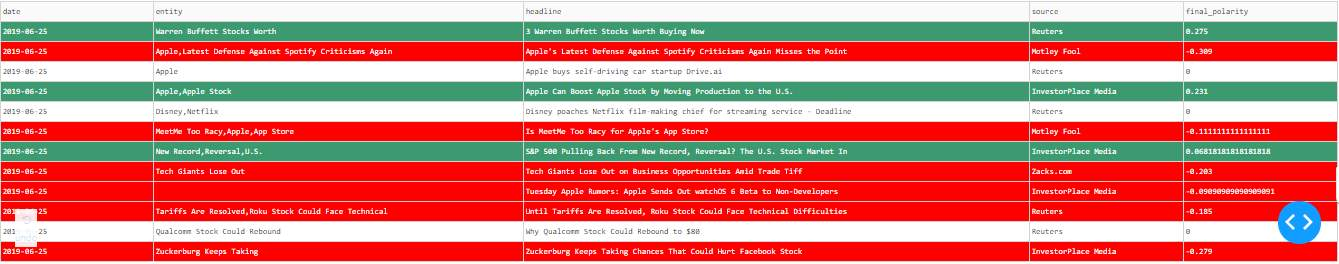The final tab is the prediction summary tab which represents the prediction information we have done so far. It had a line graph representing the stock prices and their close prices. The double vertical bar graph represents the positive and negative counts of all the days. The table at last represents the complete prediction and result summary.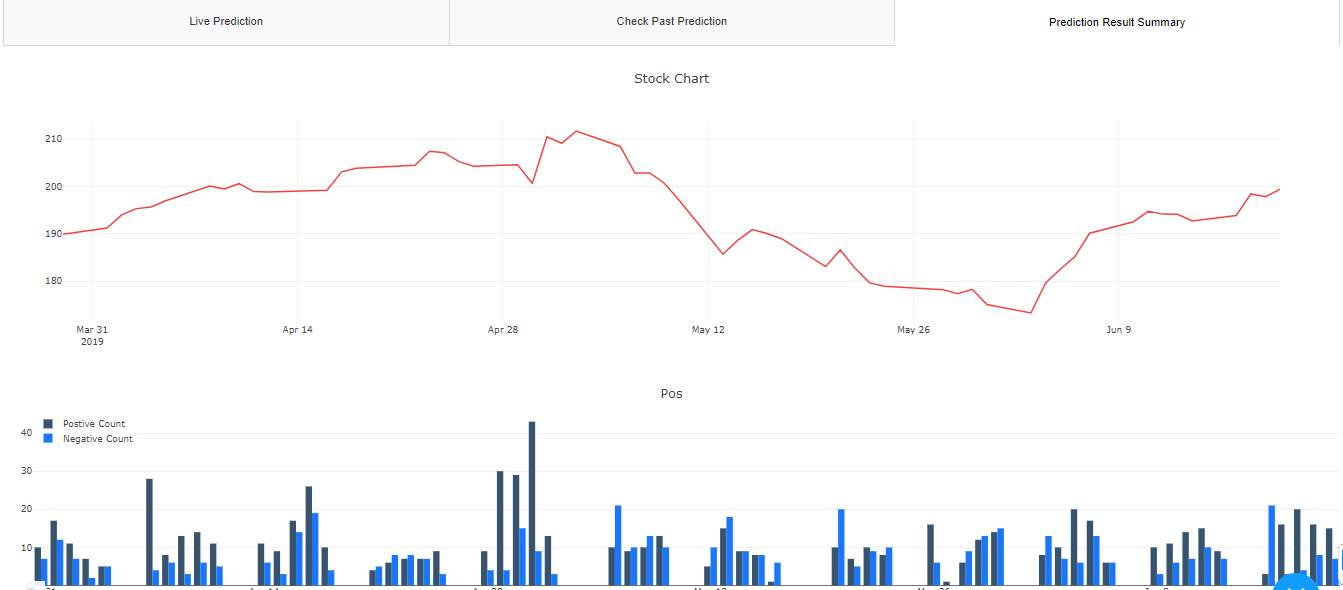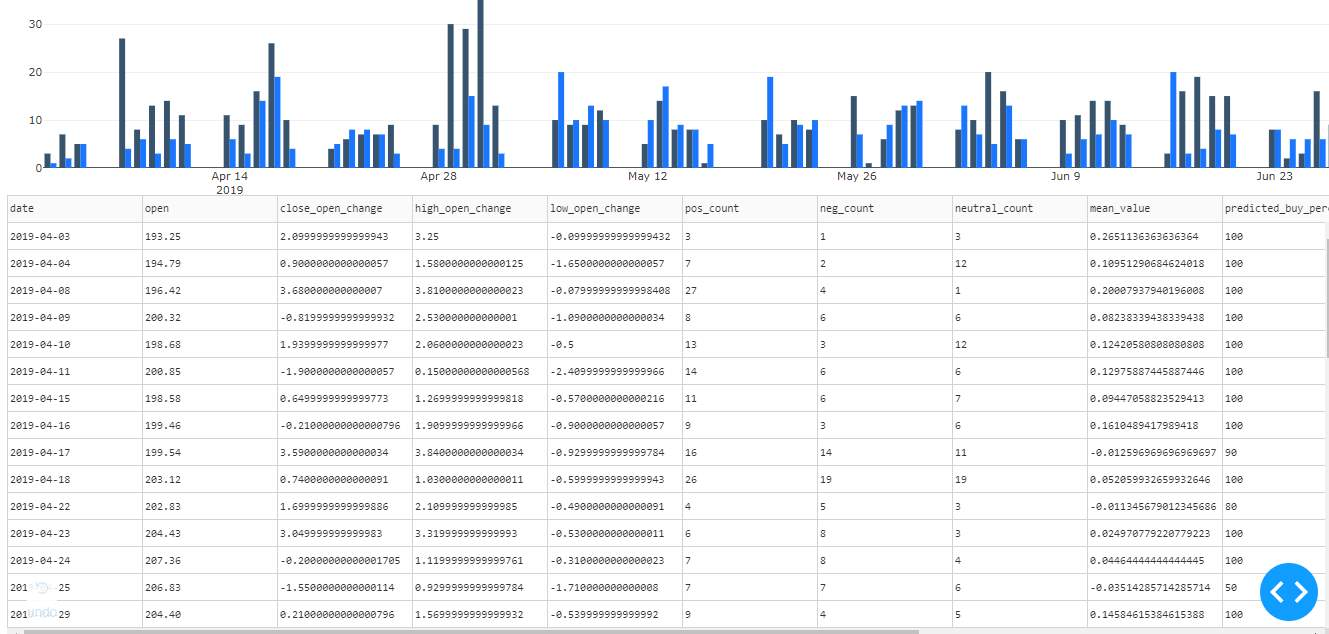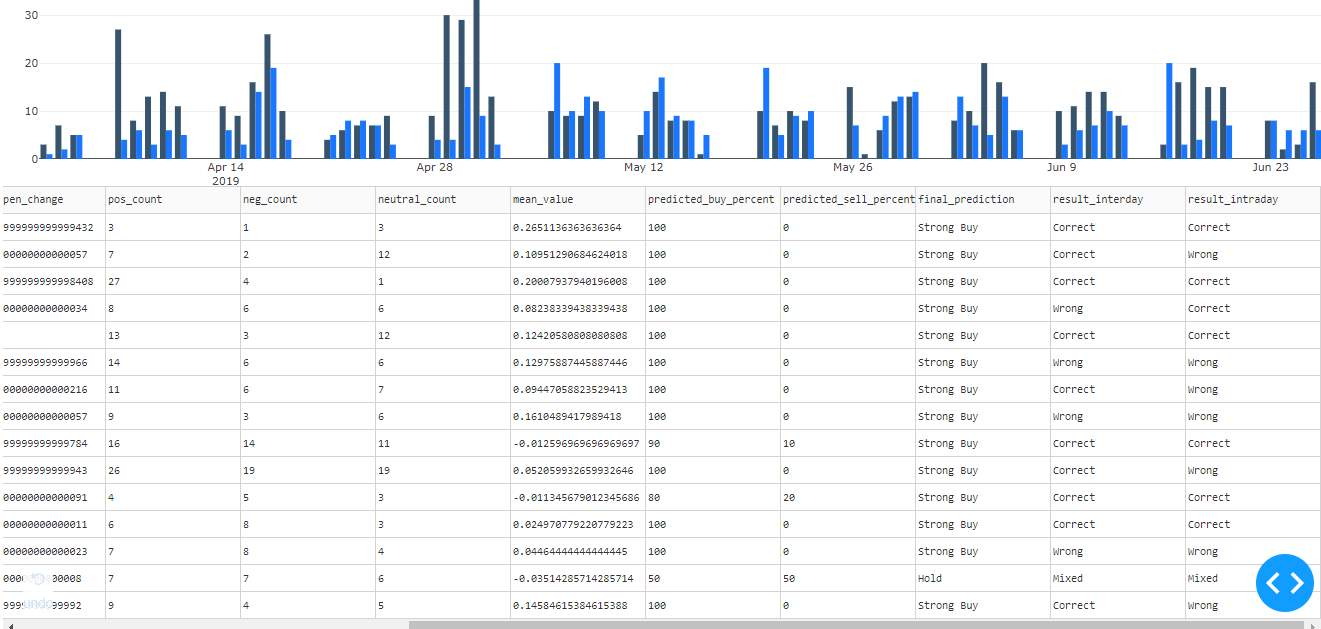Conclusion and Future Scope

With more and more advancements in machine learning techniques used for stock price change predictions, several distant factors effecting the stock market are able to be traced for a better control over an individual’s financial investments. Also the information about the major and minor incidents relating to an organization its relation with the organizations financial value can be thus established.

I would further like to increase the accuracy obtained from this project by implementing nuances of procedures and API’s for generating sentiment for the headlines.

As we are using only the headlines for this prediction, another scope would be to increase the domain by giving the news articles also as input for analysis.

Working code

import requests

import pandas as pd

import psycopg2

from scrapy.selector import Selector

import datetime

final_df = pd.DataFrame()

url = ‘https://finviz.com/quote.ashx?t=’ + symbol

page = requests.get(url)

sel = Selector(text=page.content)

times = sel.xpath(‘//table[contains(@id,”news-table”)]/tr/td/text()’).extract()

sources = sel.xpath(‘//table[contains(@id,”news-table”)]/tr/td/span/text()’).extract()

times = [x.strip() for x in times if x.strip()!=”]

count = 0

if len(times[i]) > 10:

count += 1

if count == number_of_days + 1:

break

source = sources[i]

final_df = final_df.append(df)

return final_df

final_df = pd.DataFrame()

for j in range(1, pages):

page = requests.get(url)

sel = Selector(text=page.content)

times = [x.strip() for x in times if x.strip()!=”]

len_sources = len(sources)

source_c = 0

time = times[i].strip()

date_index = time.find(‘-‘)

date = datetime.datetime.strptime(time[:date_index – 12].strip(), “%m/%d/%Y”).date()

if len(time[22:])==0:

source = sources[source_c]

source_c += 1

else:

source = “Reuters”

df = pd.DataFrame({‘date’: date, ‘name’: ‘Apple’, ‘symbol’: ‘AAPL’, ‘exchange’: ‘NASDAQ’,

final_df = final_df.append(df)

final_df = final_df.sort_values([‘date’])

return final_df

import pandas as pd

import psycopg2

import nltk

import re

from nltk import ne_chunk, pos_tag, word_tokenize

from nltk.tree import Tree

def get_continuous_chunks(text

chunked = ne_chunk(pos_tag(word_tokenize(text)))

continuous_chunk = []

current_chunk = []

for i in chunked:

if type(i) == Tree:

current_chunk.append(” “.join([token for token, pos in i.leaves()]))

elif current_chunk:

named_entity = ” “.join(current_chunk)

if named_entity not in continuous_chunk:

continuous_chunk.append(named_entity)

current_chunk = []

else:

continue

return continuous_chunk

letters_only = re.sub(” d+”, ” “, letters_only)

return “”.join(letters_only)

process_headline = review_to_words(sentence)#removing special charecters and numbers.

words = get_continuous_chunks(sentence)#entities and tree.

count = 1

clean_df = pd.DataFrame()

rows = rows

date = rows[‘date’]

source = rows[“source”]

count += 1

df = pd.DataFrame({‘date’: date, ‘name’: “Apple”, ‘symbol’: ‘AAPL’, ‘exchane’: ‘NASDAQ’,

clean_df = clean_df.append(df)

return clean_df

import nltk

import textblob

import nltk.sentiment

import pandas as pd

import psycopg2

def get_simple_polarity(sentence):

tokenizer = nltk.tokenize.treebank.TreebankWordTokenizer() #split standard contractions,punctuations.

pos_words = 0

neg_words = 0

neutral = 0

tokenized_sent = [word.lower() for word in tokenizer.tokenize(sentence)]

if len(tokenized_sent) == 0:

return 0

opinion_lexicon = nltk.corpus.opinion_lexicon

for word in tokenized_sent:

if word in opinion_lexicon.positive():

pos_words += 1

elif word in opinion_lexicon.negative():

neg_words += 1

else:

neutral += 1

total_words = pos_words + neg_words + neutral#to neutralize the polarity.

polarity = (pos_words – neg_words)/total_words

return polarity

def get_textblob_polarity(sentence):

analysis = textblob.TextBlob(sentence)

return analysis.sentiment.polarity

polarity = 0

pos = “pos”

neg = “neg”

return polarity

def get_final_polarity(sentence):

simple_polarity = get_simple_polarity(sentence)

blob_polarity = get_textblob_polarity(sentence)

review = 0

pos = “pos”

neg = “neg”

else:

if blob_polarity == 0:

review = simple_polarity

else:

review = blob_polarity

return review

final_df = pd.DataFrame()

for rows in clean_df.iterrows():

try:

rows = rows

date = rows[“date”]

symbol = rows[“symbol”]

exchange = rows[“exchange”]

source = rows[“source”]

entity = rows[“entity”]

‘entity’:entity,’source’: source, ‘simple_polarity’: simple_polarity, ‘textblob_polarity’:textblob_polarity,

columns=[‘date’, ‘symbol’, ‘exchange’, ‘headline’, ‘source’, ‘entity’, ‘simple_polarity’,

print(date)

final_df = final_df.append(polarity_df)

except Exception as e:

print(e)

continue

return final_df

import pandas as pd

import psycopg2

import math

import datetime

def get_polarity_count(final_polarity_list):

pos_count = 0

neg_count = 0

neutral_count = 0

for polarity in final_polarity_list:

if polarity < 0 :

neg_count += 1

elif polarity > 0:

pos_count += 1

else:

neutral_count += 1

return pos_count, neg_count, neutral_count

final_polarity_list = polarity_df[‘final_polarity’].to_list()

if len(polarity_df)!= 0:

pos_count, neg_count, neutral_count = get_polarity_count(final_polarity_list)

polarity_df = polarity_df.loc[polarity_df[‘final_polarity’] != 0.0]

mean_value = polarity_df[‘final_polarity’].mean()

if math.isnan(mean_value):

mean_value = 0.0

else:

mean_value = 0.0

pos_count = 0

neg_count = 0

neutral_count = 0

final_df = pd.DataFrame({‘date’:date, ‘symbol’:’AAPL’, ‘exchange’:’NASDAQ’,’mean_value’:mean_value,’pos_count’:pos_count,

‘neg_count’:neg_count,’neutral_count’:neutral_count},index=)

final_df[‘p_10’] = 0.9 * sentiment_df[‘p_10’] + 0.1 * mean_value

final_df[‘p_20’] = 0.8 * sentiment_df[‘p_20’] + 0.2 * mean_value

final_df[‘p_30’] = 0.7 * sentiment_df[‘p_30’] + 0.3 * mean_value

final_df[‘p_40’] = 0.6 * sentiment_df[‘p_40’] + 0.4 * mean_value

final_df[‘p_50’] = 0.5 * sentiment_df[‘p_50’] + 0.5 * mean_value

final_df[‘p_60’] = 0.4 * sentiment_df[‘p_60’] + 0.6 * mean_value

final_df[‘p_70’] = 0.3 * sentiment_df[‘p_70’] + 0.7 * mean_value

final_df[‘p_80’] = 0.2 * sentiment_df[‘p_80’] + 0.8 * mean_value

final_df[‘p_90’] = 0.1 * sentiment_df[‘p_90’] + 0.9 * mean_value

return final_df

import pandas as pd

import psycopg2

from collections import Counter

def generate_all_recommendation(sentiment_df, stock_df):

sentiment_df = sentiment_df[[‘date’, ‘pos_count’, ‘neg_count’, ‘neutral_count’,

‘mean_value’, ‘p_10’, ‘p_20’, ‘p_30’, ‘p_40’, ‘p_50’, ‘p_60’, ‘p_70’,

‘p_80’, ‘p_90’]]

merge_df = pd.merge(stock_df,sentiment_df,on=”date”, how=”right”)

merge_df = merge_df.sort_values([‘date’])

merge_df = merge_df.fillna(method=’ffill’)

final_df = pd.DataFrame()

for rows in merge_df.iterrows():

rows = rows

date = rows[‘date’]

symbol = rows[‘symbol’]

exchange = rows[‘exchange’]

open_price = rows[‘open’]

high_price = rows[‘high’]

close_price = rows[‘close’]

low_price = rows[‘low’]

pos_count = rows[‘pos_count’]

neg_count = rows[‘neg_count’]

neutral_count = rows[‘neutral_count’]

mean_value = rows[‘mean_value’]

change = float(rows[‘close’]) – float(rows[‘open’])

result = []

for i in range(10,20):

if rows[i] > 0 and change > 0 :

elif rows[i] > 0 and change < 0:

if rows[i] < 0 and change < 0 :

result.append(“sell”)

elif rows[i] < 0 and change > 0:

result.append(“sell”)

result_dict = Counter(result)

sell_percent = (int(result_dict[‘sell’])/10) * 100

elif sell_percent >= 80.0:

final_prediction = ‘Strong Sell’

elif sell_percent < 80.0 and sell_percent > buy_percent:

final_prediction = ‘Sell’

final_prediction = “Hold”

df = pd.DataFrame({‘date’:date, ‘symbol’: symbol, ‘exchange’: exchange, ‘open’: open_price, ‘high’: high_price,

‘low’: low_price, ‘close’:close_price, ‘change’:change, ‘mean_value’:mean_value,’pos_count’:pos_count,

‘predicted_sell_percent’: sell_percent, ‘final_prediction’:final_prediction},index=)

final_df = final_df.append(df)

return final_df

def generate_today_recommendation(sentiment_df, stock_df):

open_price = stock_df[‘open’].iloc

high_price = stock_df[‘high’].iloc

close_price = stock_df[‘close’].iloc

low_price = stock_df[‘low’].iloc

final_df = pd.DataFrame()

for rows in sentiment_df.iterrows():

rows = rows

date = rows[‘date’]

neutral_count = rows[‘neutral_count’]

symbol = rows[‘symbol’]

exchange = rows[‘exchange’]

pos_count = rows[‘pos_count’]

neg_count = rows[‘neg_count’]

mean_value = rows[‘mean_value’]

change = float(close_price) – float(open_price)

result = []

for i in range(6,16):

if rows[i] > 0 and change > 0 :

elif rows[i] > 0 and change < 0:

if rows[i] < 0 and change < 0 :

result.append(“sell”)

elif rows[i] < 0 and change > 0:

result.append(“sell”)

result_dict = Counter(result)

sell_percent = (int(result_dict[‘sell’])/10) * 100

elif sell_percent >= 80.0:

final_prediction = ‘Strong Sell’

elif sell_percent < 80.0 and sell_percent > buy_percent:

final_prediction = ‘Sell’

final_prediction = “Hold”

df = pd.DataFrame({‘date’:date, ‘symbol’: symbol, ‘exchange’: exchange, ‘open’: open_price, ‘high’: high_price,

‘low’: low_price, ‘close’:close_price, ‘change’:change, ‘mean_value’:mean_value,’pos_count’:pos_count,

‘predicted_sell_percent’: sell_percent, ‘final_prediction’:final_prediction},index=)

final_df = final_df.append(df)

return final_df

def generate_result_summary(date, stock_df, recommendation_df):

df = pd.DataFrame()

date = stock_df[‘date’].iloc

open_price = stock_df[‘open’].iloc

close_price = stock_df[‘close’].iloc

high_price = stock_df[‘high’].iloc

symbol = recommendation_df[‘symbol’].iloc

low_price = stock_df[‘low’].iloc

exchange = recommendation_df[‘exchange’].iloc

neg_count = recommendation_df[‘neg_count’].iloc

pos_count = recommendation_df[‘pos_count’].iloc

mean_value = recommendation_df[‘mean_value’].iloc

neutral_count = recommendation_df[‘neutral_count’].iloc

sell_percent = recommendation_df[‘predicted_sell_percent’].iloc

final_prediction = recommendation_df[‘final_prediction’].iloc

result_interday = ”

close_open_change = float(close_price) – float(open_price)

high_open_change = float(high_price) – float(open_price)

low_open_change = float(low_price) – float(open_price)

one_percent = float(open_price)/100

print(“One Percent”, one_percent)

if final_prediction == “Hold”:

result_interday = “Mixed”

if close_open_change > 0:

result_interday = ‘Correct’

else:

result_interday = ‘Wrong’

if high_open_change > 0 :

else:

elif final_prediction == ‘Strong Buy’ :

if close_open_change > 0:

result_interday = ‘Correct’

else:

result_interday = ‘Wrong’

if high_open_change > one_percent :

else:

elif final_prediction == ‘Sell’ :

if close_open_change < 0:

result_interday = ‘Correct’

else:

result_interday = ‘Wrong’

if abs(low_open_change) > 0 :

else:

elif final_prediction == ‘Strong Sell’ :

if close_open_change < 0:

result_interday = ‘Correct’

else:

result_interday = ‘Wrong’

if abs(low_open_change) > one_percent :

else:

df = pd.DataFrame({‘date’:date, ‘symbol’: symbol, ‘exchange’: exchange, ‘open’: open_price, ‘close_open_change’: close_open_change,

‘high_open_change’: high_open_change, ‘low_open_change’:low_open_change, ‘mean_value’:mean_value,’pos_count’:pos_count,

return df

import pandas as pd

import psycopg2

import datetime

conn = psycopg2.connect(database=”test”, user = “postgres”, password = “test123”, host = “127.0.0.1”, port = “5432”)

sql_command = “SELECT * FROM result_headline_data ;”

df.to_csv(‘result.csv’)

conn.close()

correct_interday = len(df.loc[df[‘result_interday’] == ‘Correct’])

wrong_interday = len(df.loc[df[‘result_interday’] == ‘Wrong’])

total_interday = correct_interday + wrong_interday

accuracy_interday = (correct_interday/total_interday) * 100

print(“Correct Interday Count”,correct_interday)

print(“Wrong Interday Count”,wrong_interday)

print(“Accuracy Interday”,accuracy_interday)

References

View all

## DMCA / Removal Request

If you are the original writer of this essay and no longer wish to have your work published on UKEssays.com then please:

Related Services

Prices from

KD47

Approximate costs for:

• 1000 words
• 7 day deliveryHumanity University

Dedicated to your worth and value as a human being!

Related Lectures